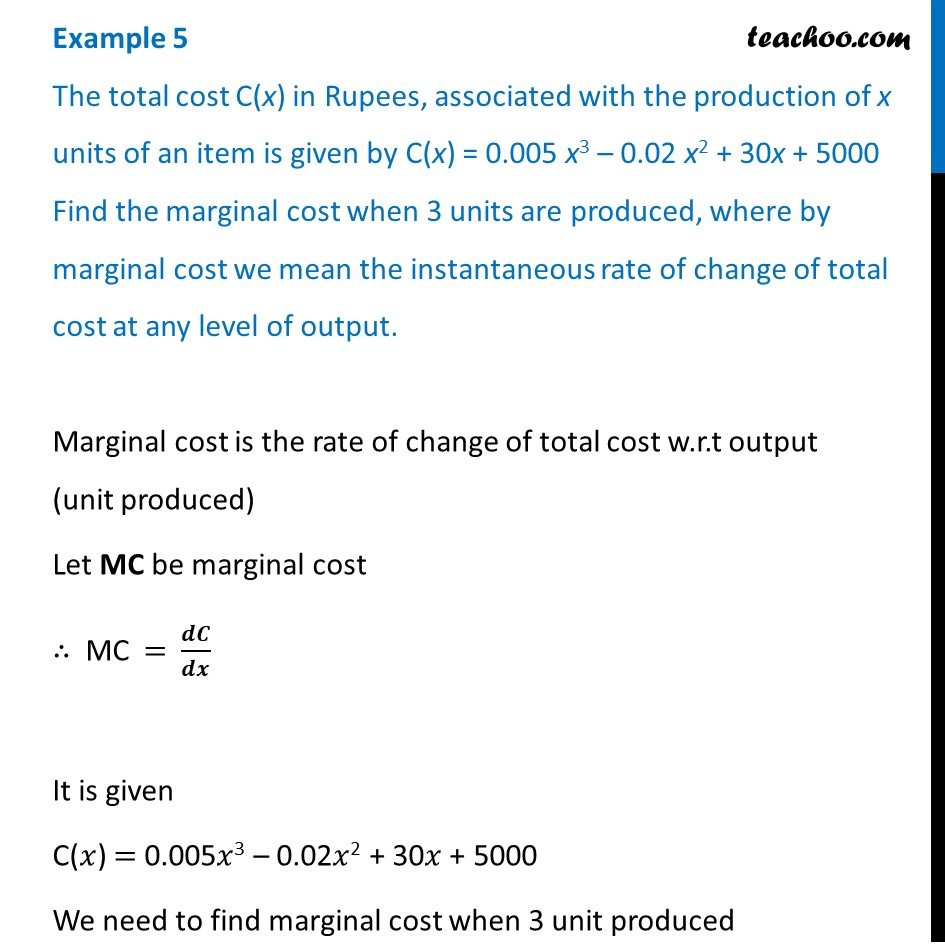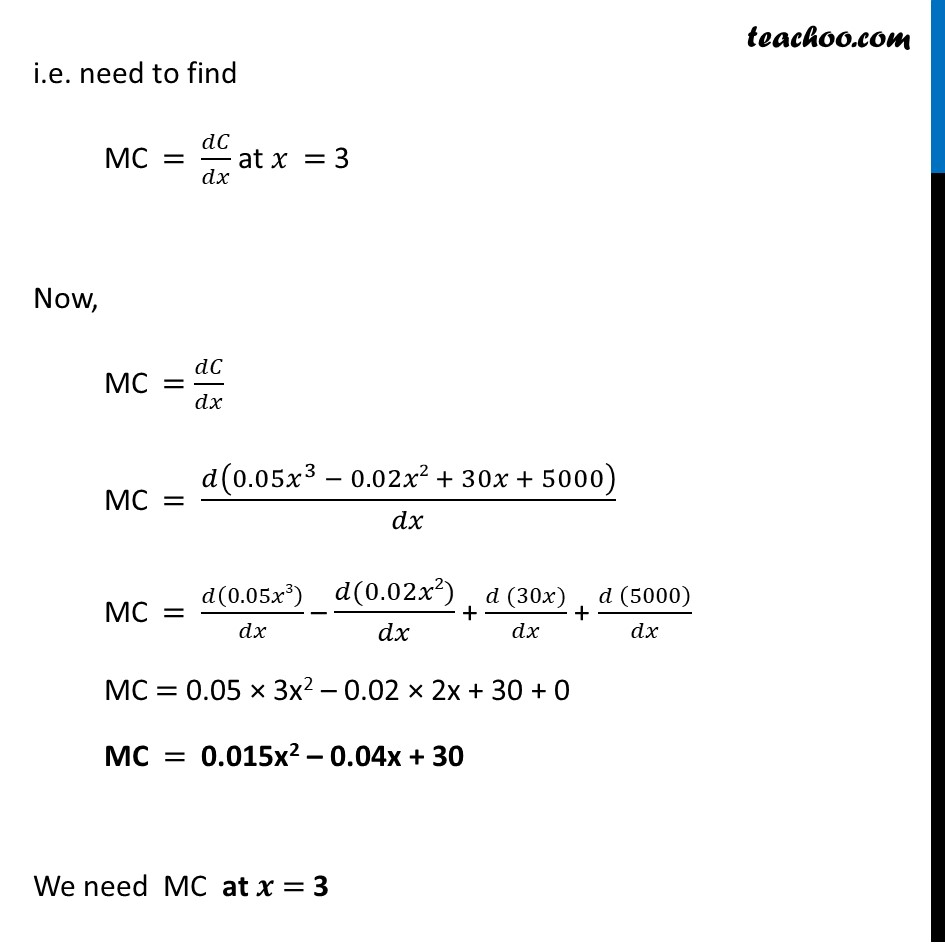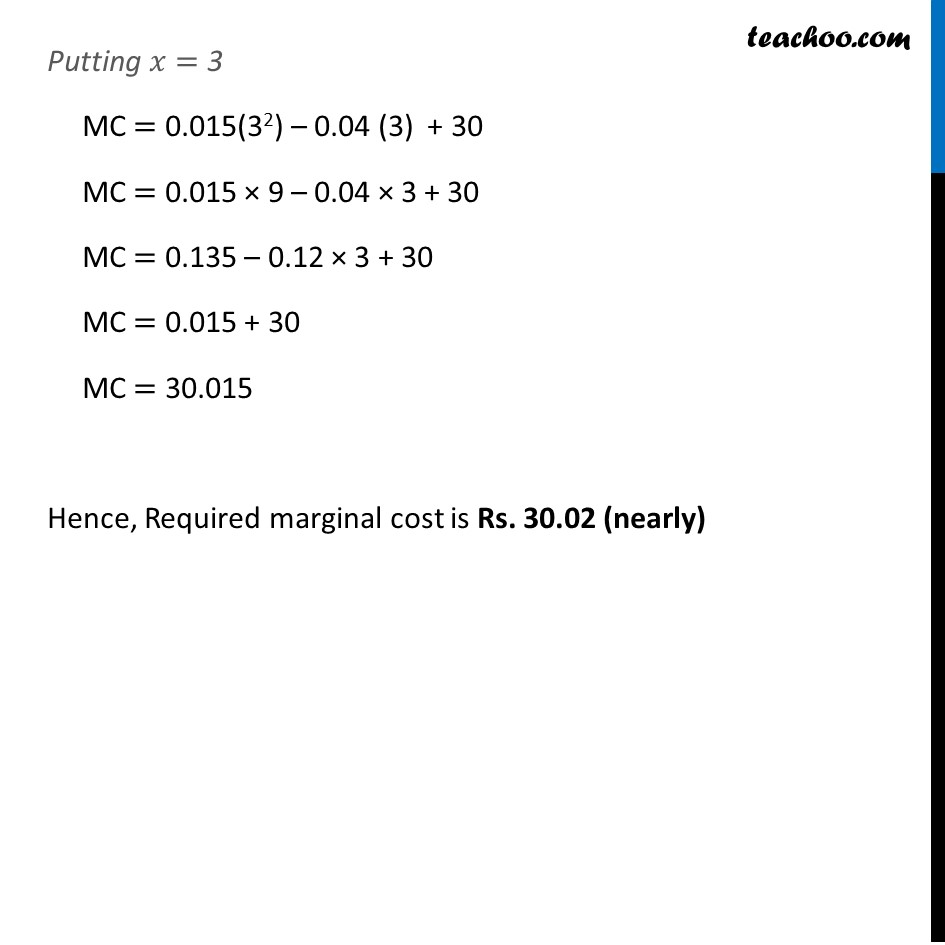Examples

Chapter 6 Class 12 Application of Derivatives
Serial order wiseLearn in your speed, with individual attention - Teachoo Maths 1-on-1 Class

### Transcript

Example 5 The total cost C(x) in Rupees, associated with the production of x units of an item is given by C(x) = 0.005 x3 – 0.02 x2 + 30x + 5000 Find the marginal cost when 3 units are produced, where by marginal cost we mean the instantaneous rate of change of total cost at any level of output.Marginal cost is the rate of change of total cost w.r.t output (unit produced) Let MC be marginal cost ∴ MC = 𝒅𝑪/𝒅𝒙 It is given C(𝑥) = 0.005𝑥3 – 0.02𝑥2 + 30𝑥 + 5000 We need to find marginal cost when 3 unit produced i.e. need to find MC = 𝑑𝐶/𝑑𝑥 at 𝑥 = 3 Now, MC = 𝑑𝐶/𝑑𝑥 MC = 𝑑(0.05𝑥^3 − 0.02𝑥2 + 30𝑥 + 5000)/𝑑𝑥 MC = 𝑑(0.05𝑥3)/𝑑𝑥 – (𝑑(0.02𝑥2))/𝑑𝑥 + (𝑑 (30𝑥))/𝑑𝑥 + (𝑑 (5000))/𝑑𝑥 MC = 0.05 × 3x2 – 0.02 × 2x + 30 + 0 MC = 0.015x2 – 0.04x + 30 We need MC at 𝒙 = 3 Putting 𝑥= 3 MC = 0.015(32) – 0.04 (3) + 30 MC = 0.015 × 9 – 0.04 × 3 + 30 MC = 0.135 – 0.12 × 3 + 30 MC = 0.015 + 30 MC = 30.015 Hence, Required marginal cost is Rs. 30.02 (nearly)